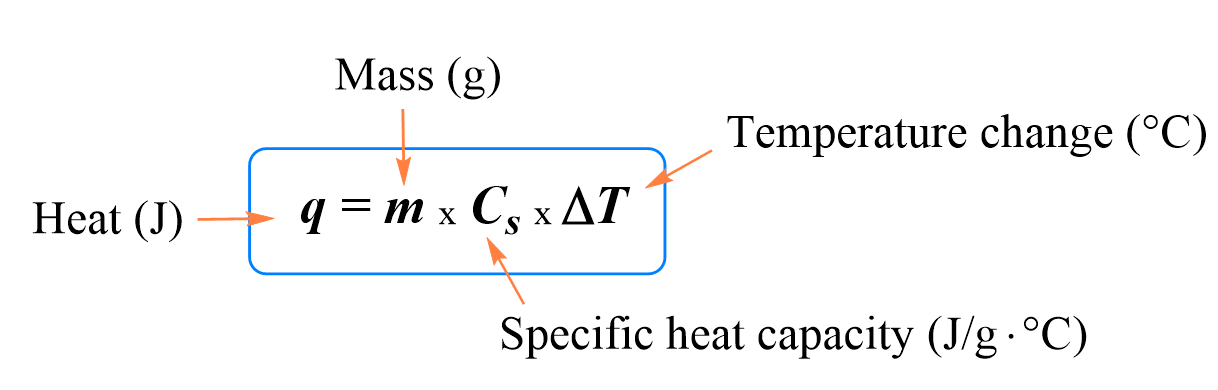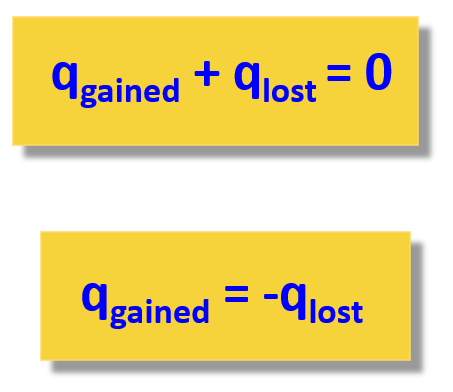## General Chemistry

In the previous post, we talked about heat capacity, specific heat, and the formula for the relationship between these and the change in temperature.Below are some additional practice examples of heat capacity problems.

When solving a problem related to heat capacity and heat transfer, remember that most of the time, it is assumed the heat is not lost, and it only flows from the object with a higher temperature to the colder one:The second expression adding the lost and gained heat is easier to use because you do not need to remember which one has a negative sign.

#### Practice

1.

How much heat does it take to increase the temperature of a 540.6-g sample of Fe from 20.0 °C to 84.3 °C? The specific heat of iron = 0.450 J/g °C.

15.6 kJ

Solution

A similar question to that in problem 8, and we are going to use the following equation:

q = m × Cs × ΔT

Where, q is the amount of heat in J, m is the mass, Cs is the specific heat capacity of the material in J/g oC , and ΔT is the change in temperature (Tfinal – Tinitial). Remember, the specific heat is the quantity of heat energy necessary to increase the temperature of 1 g of a substance by 1°C.

All we need to do is to plug the numbers into the equation of the heat:

${\rm{q}}\;{\rm{ = }}\;{\rm{540}}{\rm{.6}}\;\cancel{{\rm{g}}}\;{\rm{ \times }}\;\frac{{{\rm{0}}{\rm{.450}}\;{\rm{J}}}}{{\cancel{{\rm{g}}}\;\cancel{{^{\rm{o}}{\rm{C}}}}}}\;{\rm{ \times }}\;{\rm{(84}}{\rm{.3}}\;{\rm{ – }}\;{\rm{20}}{\rm{.0)}}\;\cancel{{^{\rm{o}}{\rm{C}}}}\;{\rm{ = }}\;{\rm{15}}{\rm{.6}}\;{\rm{ \times }}\;{\rm{1}}{{\rm{0}}^{{\rm{3}}\;}}{\rm{J}}$

To convert the J to kJ, we need to divide it by 1,000, so it is 15.6 kJ

2.

Calculate the specific heat capacity of a metal if a 17.0 g sample requires 481 J to change the temperature of the metal from 25.0 °C to 67.0 °C?

0.674 J/g°C

Solution

We are going to rearrange the following equation for the relation of heat, specific heat capacity, and temperature change:

q = m × C × ΔT

${\rm{C}}\;{\rm{ = }}\;\frac{{\rm{q}}}{{{\rm{m}}\;{\rm{\Delta T}}}}$

${\rm{C}}\;{\rm{ = }}\;\frac{{{\rm{481}}\;{\rm{J}}}}{{{\rm{17}}{\rm{.0}}\;{\rm{g}}\;{\rm{(67}}{\rm{.0}}\;{\rm{ – }}\;{\rm{25}}{\rm{.0)}}{\;^{\rm{o}}}{\rm{C}}}}\;{\rm{ = }}\;\frac{{{\rm{0}}{\rm{.674}}\;{\rm{J}}}}{{{\rm{g}}{\;^{\rm{o}}}{\rm{C}}}}\;$

3.

Calculate the energy of combustion for one mole of butane if burning a 0.367 g sample of butane (C4H10) has increased the temperature of a bomb calorimeter by 7.73 °C. The heat capacity of the bomb calorimeter is 2.36 kJ/ °C.

2,875 kJ/mol

Solution

Because the combustion of butane increased the temperature of a bomb calorimeter, it is an exothermic reaction. This means, the heat goes from the reaction to the calorimeter and assuming there is no heat loss, the heat released by the reaction is equal to the heat absorbed by the calorimeter:

-qreaction  = qcal

Since the reaction occurs under conditions of constant volume, qrxn = ΔErxn, so we need to calculate the qcal using the following formula:

qcal = Ccal x ΔT

qcal = 2.36 kJ/ °C x 7.73 °C = 18.2 kJ

Therefore, qrxn = -18.2 kJ

This is the change in the internal energy of the reaction for that specific amount of butane that was burned.

To get ΔErxn per mole of butane, we need to divide qrxn by the number of moles that actually reacted. To find the number of moles, we use the mas and molar mass of butane:

n(C4H10) = 0.367 g/58.0 g/mol = 0.00633 mol

And after this, we divide the heat of the reaction by the number of moles of butane, to get the heat of the reaction per mole of butane:

ΔErxn = 18.2 kJ/0.00633 mol = 2.88 x 103 kJ/mol

Alternatively, we can set up a cross-multiplication correlation:

0.00633 mol C4H10 – 18.2 kJ

1 mol C4H10 – X kJ

X = 2.88 x 103 kJ/mol

4.

How many joules of energy is required to melt 40.0 g of ice at 0 °C? The heat of fusion (ΔHfus) for ice is 334.0 J/g.

13,360 J

Solution

Since the ice/water is already at 0 oC (melting/freezing temperature), the added heat does not change the temperature of the ice and water mixture, but rather it is used for the transition from solid to liquid.

To calculate the amount of energy required to achieve a state change, in this case melting, of the substance that is already at the state-change temperature, we use the following formula:

q = mΔHfus

where q is the quantity of heat energy, m is the mass, and ΔHfus is the enthalpy of fusion, sometimes called the heat of fusion. It is the energy required to fuse (melt) a gram (or a mole) of a substance.
Plugging the numbers in the formula, we get:

q = 40.0 g x 334.0 J/g = 13,360 J

Rounding off to three significant figures, we have q = 1.34 x 103 J

5.

How many kJ of energy does it take to change 36.0 g of ice at -15.0 °C to water at 0. °C ? The specific heat of ice is 2.10 J/g°C and the heat of fusion (ΔHfus) for ice is 334.0 J/g.

13.2 kJ

Solution

We mentioned in the previous problem, that since the ice/water is already at 0 oC (melting/freezing temperature), the added heat does not change the temperature of the ice and water mixture, but rather it is used for the transition from solid to liquid. This is not the case here because the ice is at -15.0 oC and before melting, it needs to be first warmed to 0 oC. Therefore, there are two stages in this process; 1) heating the ice to 0 oC, 2) melting the ice.

The overall heat of this process then is:

q = qheating + qmelting

qheating is calculated by the formula we have already used several times:

qheating = m × C × ΔT

${{\rm{q}}_{{\rm{heating}}}}\;{\rm{ = }}\;{\rm{36}}{\rm{.0}}\;\cancel{{\rm{g}}}\;{\rm{ \times }}\;\frac{{2.10\;{\rm{J}}}}{{\cancel{{\rm{g}}}\;\cancel{{^{\rm{o}}{\rm{C}}}}}}\;{\rm{ \times }}\;{\rm{(0}}\;{\rm{ – }}\;( – {\rm{15}}{\rm{.0)}}\;\cancel{{^{\rm{o}}{\rm{C}}}}\;{\rm{ = }}\;{\rm{1,134}}\;{\rm{J}}$

To calculate the amount of energy required to melt the ice, we use the following formula:

q = mΔHfus

${{\rm{q}}_{{\rm{melting}}}}\;{\rm{ = }}\;{\rm{36}}{\rm{.0}}\;\cancel{{\rm{g}}}\;{\rm{ \times }}\;\frac{{334.0\;{\rm{J}}}}{{\cancel{{\rm{g}}}\;}}\;{\rm{ = }}\;{\rm{12,024}}\;{\rm{J}}$

q = 1134 J + 12,024 J = 13,158 J

Rounding of to three significant figures, we have q = 1.32 x 104 J or 13.2 kJ

1686018818